### Home > CALC > Chapter 1 > Lesson 1.4.3 > Problem1-173

1-173.
1. For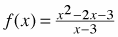and g(x) = −x + 2, find and simplify h(x) = f(g (x)) Homework Help ✎

1. Graph h(x) and write its domain in set notation.

2. Find b(x), the end behavior function of h(x).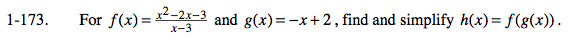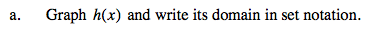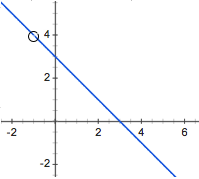D = x : x ≠ −1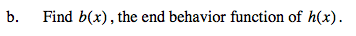The end behavior of h(x) will describe what h(x) looks like in the end (as x→∞ and as x→−∞). Holes and vertical asymptotes are ignored. Observe the graph in part (a). Notice that the graph of h(x) is almost linear. What is the equation of this line? That line, by the way, is b(x), the end behavior of h(x).